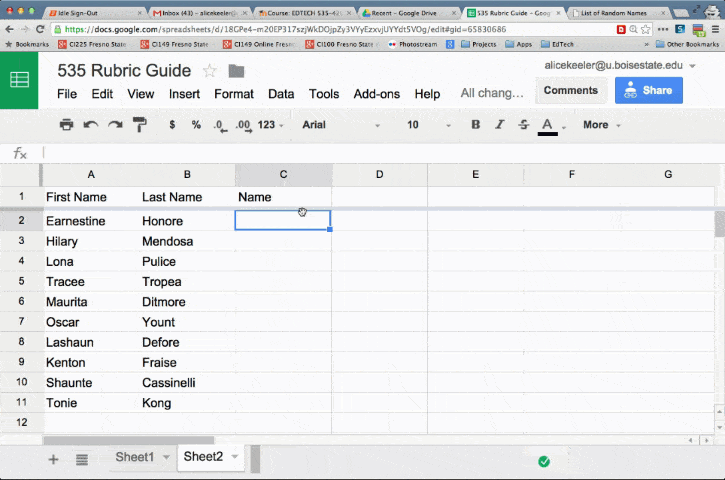Frequently I have a need to add totals to the column headers in a spreadsheet. In my budget spreadsheet, I like to have the total amount of money I’ve spent in the column header for expenses. When totalling points on assignments, I want the total at the top of the list, even though I add numbers to the bottom of the list.# Concatenate

The joining together of values in a spreadsheet is called “concatenate.” You do not need to learn this word, but it is a fun word to say 🙂

## Ampersand

Basically, you are saying AND. You want this text AND this value. The symbol for AND is the ampersand, located on the 7 key. Use the ampersand to connect the content of cells together or to join text strings to the values in cells.If you want your column header to say the word “points” and the point total in the column together in the same cell then you want to join the text “points” AND the total number of points.

Assuming you are totalling the points in column D you would use the formula:

=”points “&sum(D2:D)

When writing a formula in a spreadsheet you always start with the equals sign. Any text strings must be in quotation marks. Notice the space after the s in points. You will end up with points1000 instead of points 1000 if you forget to include the space. In the formula, you are joining the word points with the formula, sum(D2:D). In between anything you are joining together you want to use the ampersand (&).

# Example

Another example of using concatenate would be to take a student’s first name in one cell and join it with their last name in another cell. Remember you also want to join a text string of a space in between the first and last name. Equals first name AND a space AND last name.

=A2&” “&B2## Subscribe to the Alice Keeler Newsletter